# pylops.optimization.leastsquares.RegularizedInversion¶

pylops.optimization.leastsquares.RegularizedInversion(Op, Regs, data, Weight=None, dataregs=None, epsRs=None, x0=None, returninfo=False, **kwargs_solver)[source]

Regularized inversion.

Solve a system of regularized equations given the operator Op, a data weighting operator Weight, and a list of regularization terms Regs.

Parameters: Op : pylops.LinearOperator Operator to invert Regs : list Regularization operators (None to avoid adding regularization) data : numpy.ndarray Data Weight : pylops.LinearOperator, optional Weight operator dataregs : list, optional Regularization data (if None a zero data will be used for every regularization operator in Regs) epsRs : list, optional Regularization dampings x0 : numpy.ndarray, optional Initial guess returninfo : bool, optional Return info of LSQR solver **kwargs_solver Arbitrary keyword arguments for chosen solver (scipy.sparse.linalg.lsqr and pylops.optimization.solver.cgls are used as default for numpy and cupy data, respectively) xinv : numpy.ndarray Inverted model. istop : int Gives the reason for termination 1 means $$\mathbf{x}$$ is an approximate solution to $$\mathbf{d} = \mathbf{Op}\,\mathbf{x}$$ 2 means $$\mathbf{x}$$ approximately solves the least-squares problem itn : int Iteration number upon termination r1norm : float $$||\mathbf{r}||_2^2$$, where $$\mathbf{r} = \mathbf{d} - \mathbf{Op}\,\mathbf{x}$$ r2norm : float $$\sqrt{\mathbf{r}^T\mathbf{r} + \epsilon^2 \mathbf{x}^T\mathbf{x}}$$. Equal to r1norm if $$\epsilon=0$$

RegularizedOperator
Regularized operator
NormalEquationsInversion
Normal equations inversion
PreconditionedInversion
Preconditioned inversion

Notes

Solve the following system of regularized equations given the operator $$\mathbf{Op}$$, a data weighting operator $$\mathbf{W}^{1/2}$$, a list of regularization terms $$\mathbf{R}_i$$, the data $$\mathbf{d}$$ and regularization damping factors $$\epsilon_\mathbf{I}$$: and $$\epsilon_{\mathbf{R}_i}$$:

$\begin{split}\begin{bmatrix} \mathbf{W}^{1/2} \mathbf{Op} \\ \epsilon_{\mathbf{R}_1} \mathbf{R}_1 \\ \vdots \\ \epsilon_{\mathbf{R}_N} \mathbf{R}_N \end{bmatrix} \mathbf{x} = \begin{bmatrix} \mathbf{W}^{1/2} \mathbf{d} \\ \epsilon_{\mathbf{R}_1} \mathbf{d}_{\mathbf{R}_1} \\ \vdots \\ \epsilon_{\mathbf{R}_N} \mathbf{d}_{\mathbf{R}_N} \\ \end{bmatrix}\end{split}$

where the Weight provided here is equivalent to the square-root of the weight in pylops.optimization.leastsquares.NormalEquationsInversion. Note that this system is solved using the scipy.sparse.linalg.lsqr and an initial guess x0 can be provided to this solver, despite the original solver does not allow so.

## Examples using pylops.optimization.leastsquares.RegularizedInversion¶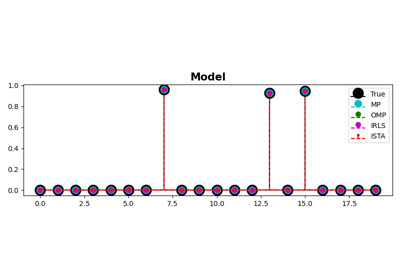MP, OMP, ISTA and FISTA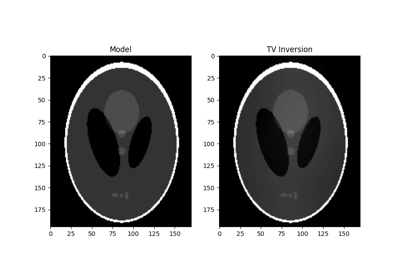Total Variation (TV) Regularization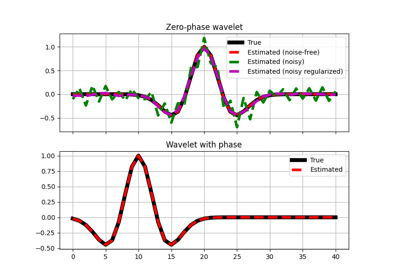Wavelet estimation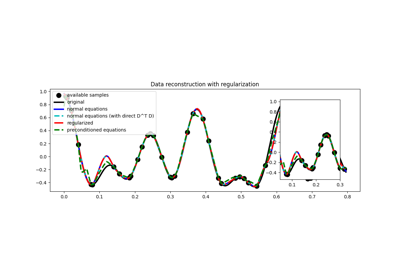03. Solvers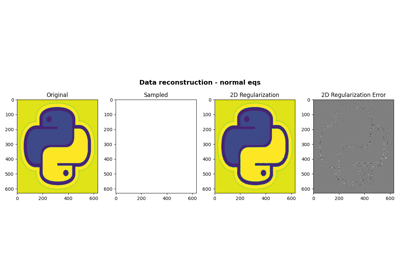06. 2D Interpolation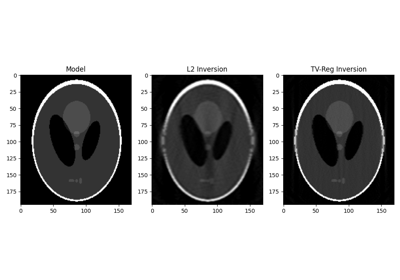16. CT Scan Imaging